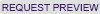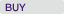# Case Detail

## Case Summary

### Practical Regression: Fixed Effects Models

Case Number: 7-112-005, Year Published: 2012

HBS Number: KEL639### Key Concepts

Economics, Market Research, Statistical Methods

### Abstract

This is the fifth in a series of lecture notes which, if tied together into a textbook, might be entitled “Practical Regression.” The purpose of the notes is to supplement the theoretical content of most statistics texts with practical advice based on nearly three decades of experience of the author, combined with over one hundred years of experience of colleagues who have offered guidance. As the title “Practical Regression” suggests, these notes are a guide to performing regression in practice.
This technical note discusses fixed effects models. Though a unified example, the note shows how omitted variable bias can plague estimates in cross-section regressions and how focusing attention on intragroup ("within") variation over time can allow for identification of regression coefficients that are free of potential bias. The note demontrates the mathematical principles behind fixed effects modeling and also explains why, in some cases, it may be preferable not to include fixed effects.

### Learning Objectives

Students will learn the following:
-How omitted variable bias can plague cross-section regression analysis
-The conceptual underpinning of fixed effects modeling
-The difference between within and between variation
-The mathematical foundations of fixed effects models
-The concept of time-invariant unobservables
-Practical tips on when and how to use fixed effects models